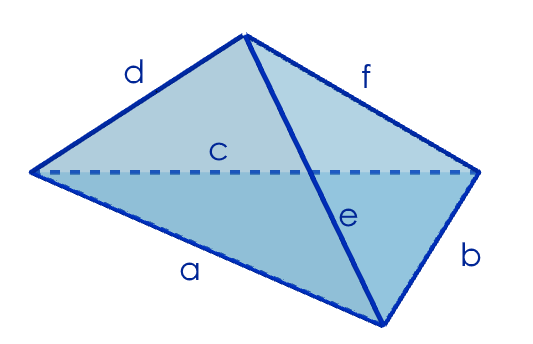#### You may also like### Overturning Fracsum

Solve the system of equations to find the values of x, y and z: xy/(x+y)=1/2, yz/(y+z)=1/3, zx/(z+x)=1/7### Rudolff's Problem

A group of 20 people pay a total of Â£20 to see an exhibition. The admission price is Â£3 for men, Â£2 for women and 50p for children. How many men, women and children are there in the group?### Polycircles

Show that for any triangle it is always possible to construct 3 touching circles with centres at the vertices. Is it possible to construct touching circles centred at the vertices of any polygon?

# Building Tetrahedra

##### Age 14 to 16Challenge Level
Sam from Bishop Strachan School in Canada used diagrams to show that the the triangles which are the faces of the tetrahedron must be congruent. Click here to see Sam's work, which also shows how it is possible to make a tetrahedron with any 4 congruent triangles.

Agathiyan from Hymers College used algebra to look for tetrahedra whose faces had perimeters of 20:First of all, I tried and got several possibilities of tetrahedra made out of four triangles with a perimeter of 20.The interesting thing was that I couldn't find a solution that doesn't use 4 congruent triangles. All my solutions had two sides of the same length. Using symbols on the provided diagram:
$a=f$
$b=d$
$c=e$

And therefore I set out to prove that it is always like this. The first thing I did was add up all the sides of the triangles, so we could get $a+b+c+d+e+f=x:$
$(a+b+c)+(a+d+e)+(b+e+f)+(c+d+f)=20+20+20+20$
$2a+2b+2c+2d+2e+2f=80$
$a+b+c+d+e+f=40$

And since $a+b+c=20$
$d+e+f=20$

Using the equations of other triangles' perimeters:
$d+e+f-(a+d+e)=20-20$ (since $a+d+e$ is the perimeter of one of the faces so must be equal to $20$)
$\Rightarrow f-a=0$
$\Rightarrow a=f$

$d+e+f-(b+e+f)=20-20$
$\Rightarrow d-b =0$
$\Rightarrow b=d$

$d+e+f-(c+d+f)=20-20$
$\Rightarrow e-c =0$
$\Rightarrow c=e$

Using these values and substituting them into the triangles:
$(a+b+c)+(a+d+e)+(b+e+f)+(c+d+f)=80$
$(a+b+c)+(a+b+c)+(b+c+a)+(c+b+a)=80$

Showing all triangles must be congruent. Also it must form a tetrahedron with the triangles sharing the same perimeter, since because they are all congruent, they share a perimeter and also the same sides, therefore all sides should perfectly tessellate in 3D, meaning the tetrahedron can be formed.

Makalp from MGIS in India and David from Singapore both looked for tetrahedra whose faces had perimeter $k$, beginning with the equations
\begin{align}a+b+c&=k&(1)\\ a+d+e&=k&(2)\\ b+e+f&=k&(3)\\ c+d+f&=k&(4)\end{align}

David used exactly the same method as Agathiyan, and the algebra is exactly the same, except that all of the $20$ s are replaced with $k$ s. This gives the exact same result that all of the triangles must be congruent. Makalp's proof is slightly different:
Subtracting equations from each other to elimate a variable and then rearranging gives the following 6 equations:
\begin{align}b+c&=d+e&(1-2)\\ a+d&=b+f&(2-3)\\ b+e&=c+d&(3-4)\\ a+c&=e+f&(1-3)&(A)\\ a+b&=d+f&(1-4)&(B)\\ a+e&=c+f&(2-4)\end{align} Subtracting $(B)$ from $(A)$ to eliminate $f$ gives \begin{align}a+c&=e+f&(A)\\ a+b&=d+f&(B)\\ c-b&=e-d&(A-B)\end{align} When this is added to equation $(1-2)$
\begin{align}b+c&=d+e &(1-2)\\ +\hspace{2mm}-b+c&=-d+e &(A-B)\\ 2c&=2e &((1-2)+(A-B))\end{align}
Therefore $c=e$.

Similarly by using the above deduced result and the equations, relationships for $a$, $b$, $d$ and $f$ were also determined.
$a=f,$ $b=d$ and $c=e$

Therefore, there are infinite possible tetrahedrons whose all 4 triangular sides have the same perimeter. All dimensions that follow the above mentioned condition is a possible solution i.e. $a=f,$ $b=d$ and $c=e.$ This, the triangular sides can be equilateral, isosceles and scalene.